# 归并排序，我举个例子你就看懂了

• 2021 年 11 月 27 日
• 本文字数：2117 字

阅读完需：约 7 分钟

## 一、算法思想

1. 将 n 个元素从中间切开，分成两部分。

2. 将剩下的数组通过递归的方式一直分割，直到数组的大小为 1，此时只有一个元素，那么该数组就是有序的了。

3. 从最底层开始逐步合并两个排好序的数列。把两个数组大小为 1 的合并成一个大小为 2 的有序数列，再把两个大小为 2 有序数列的合并成 4 的有序数列 … 直到全部小的数组合并起来。

## 三、举个栗子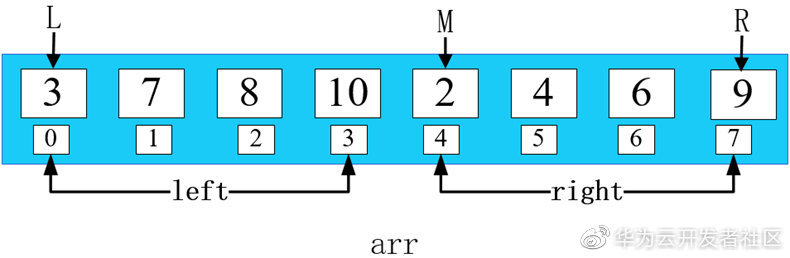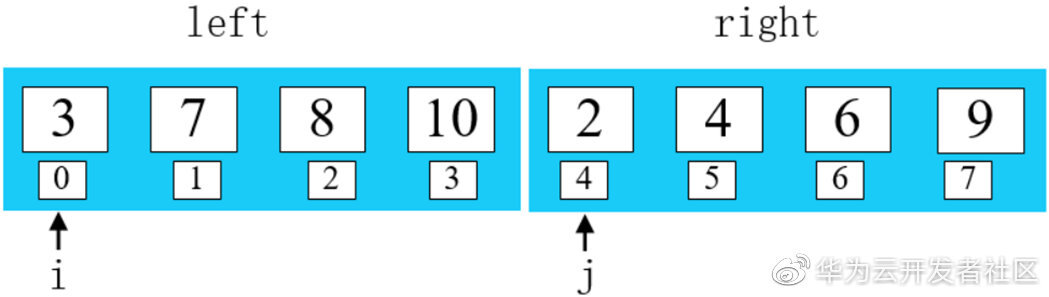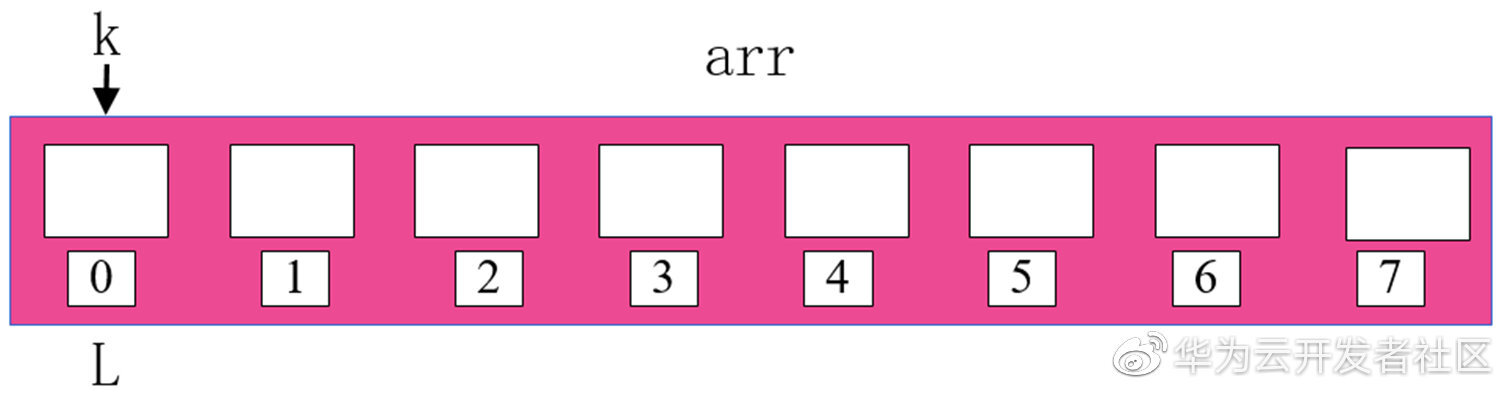2 < 3 , 将 2 填入 arr 数组 ，同时右移 j 和 k。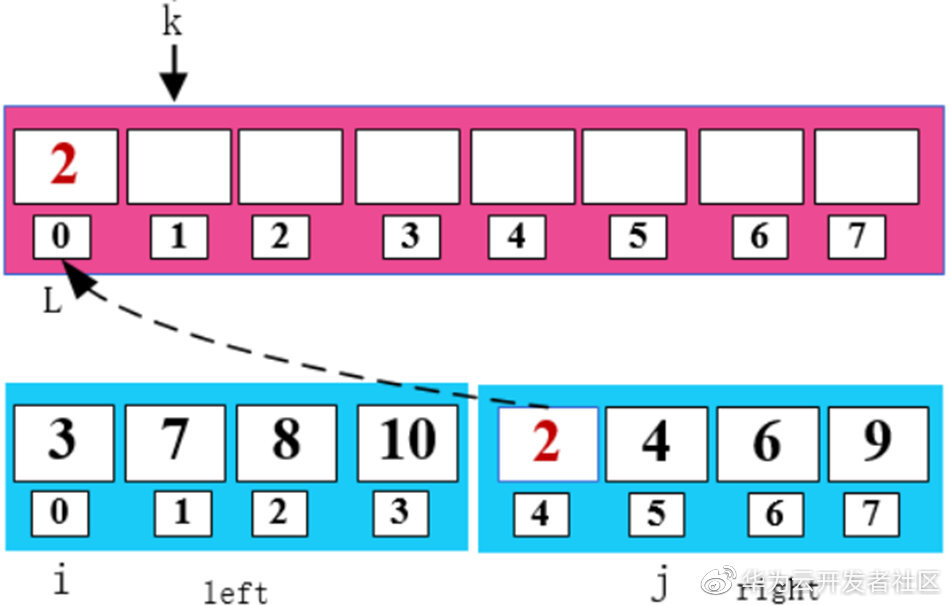​3 < 4 , 将 3 填入 arr 数组 ，同时右移 i 和 k。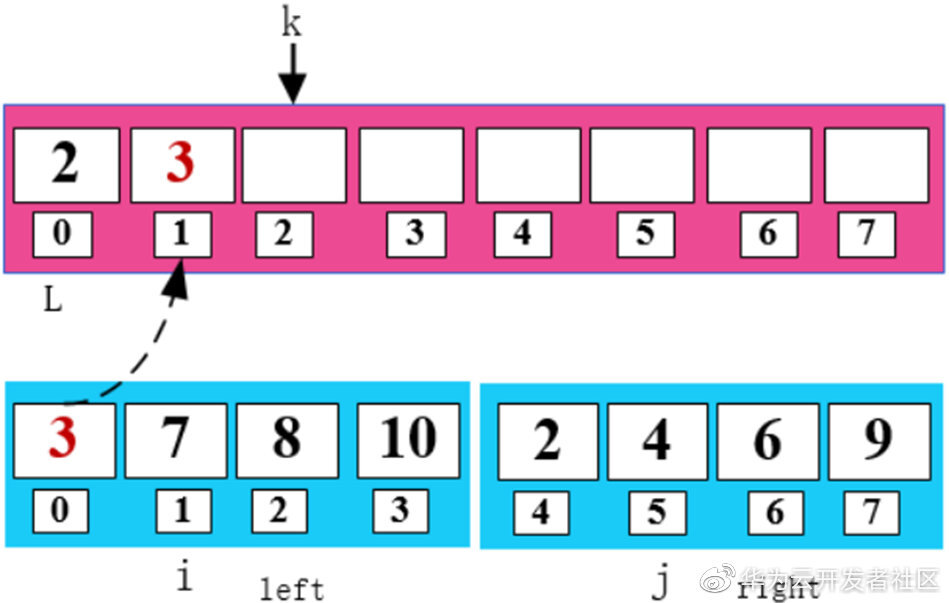​4 < 7，将 4 填入 arr 数组，同时右移 j 和 k。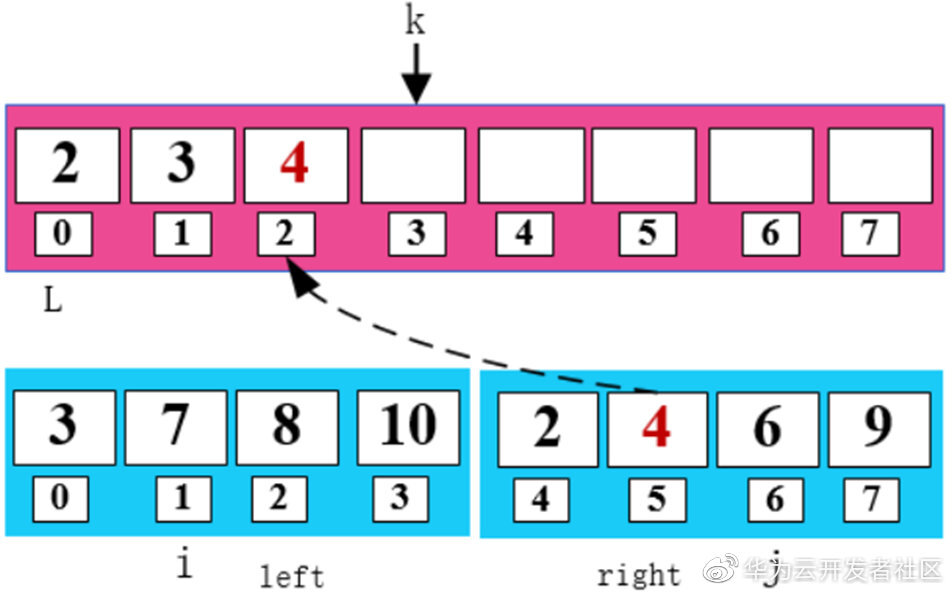​6 < 7，将 6 填入 arr 数组，同时右移 j 和 k。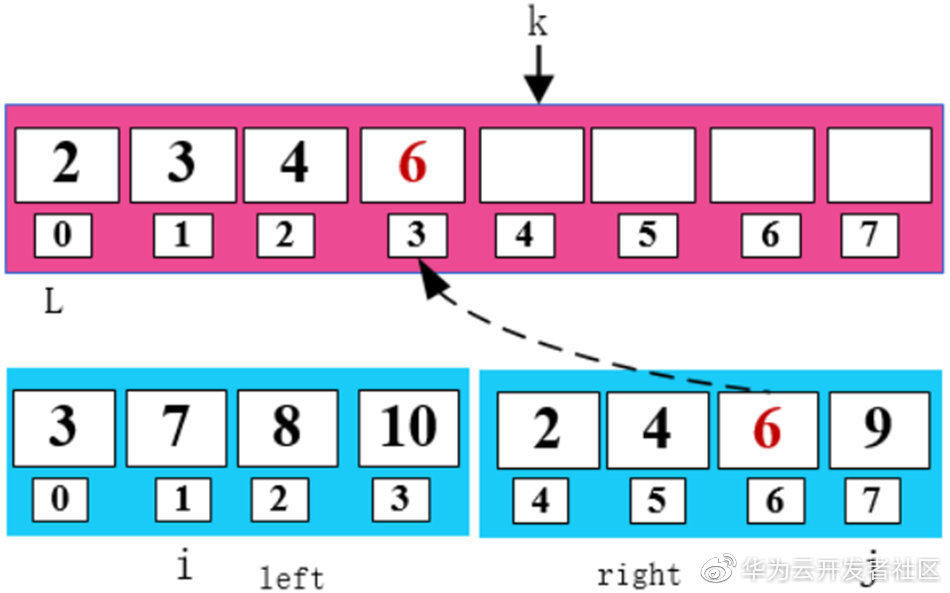​7 < 9，将 7 填入 arr 数组，同时右移 i 和 k。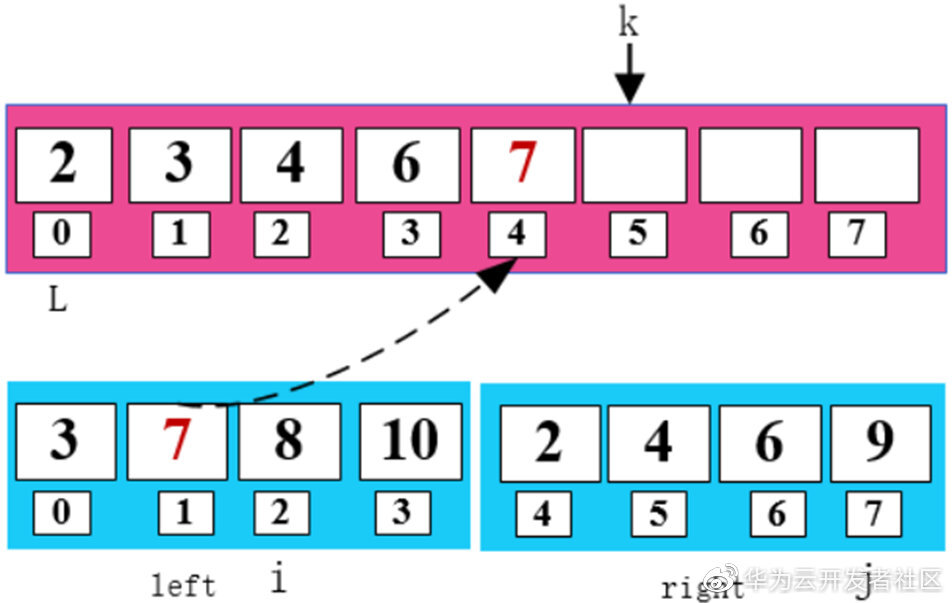​8 < 9，将 8 填入 arr 数组，同时右移 i 和 k。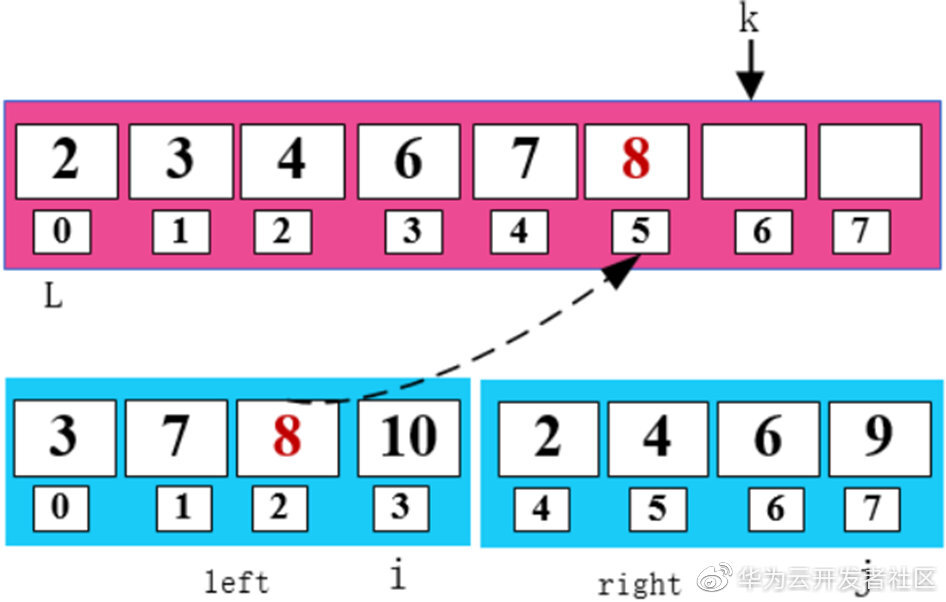​10 > 9，将 9 填入 arr 数组，同时右移 j 和 k。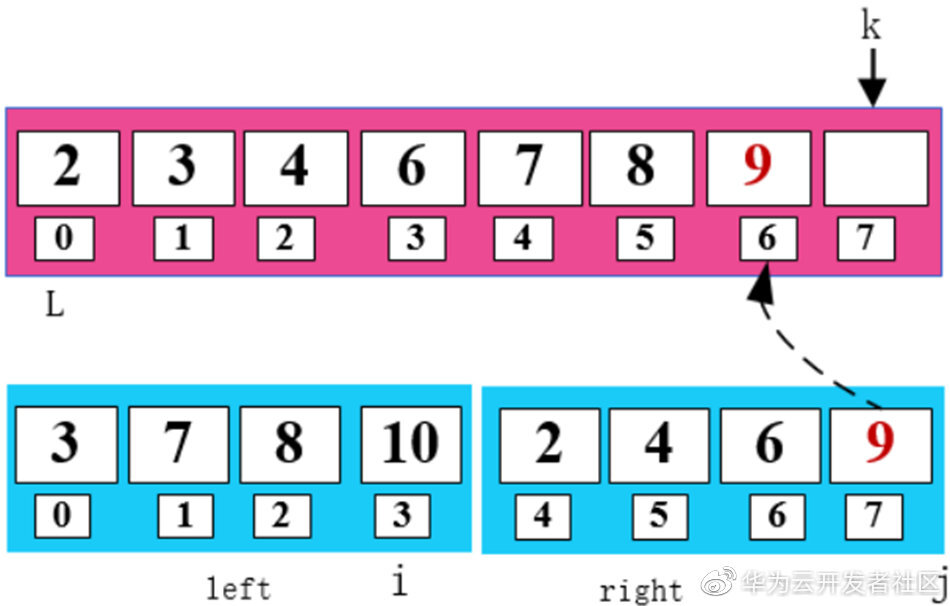​可以发现此时 right 数组已经填完了，所以此时只需要把 left 数组剩下的数字填入 arr 即可。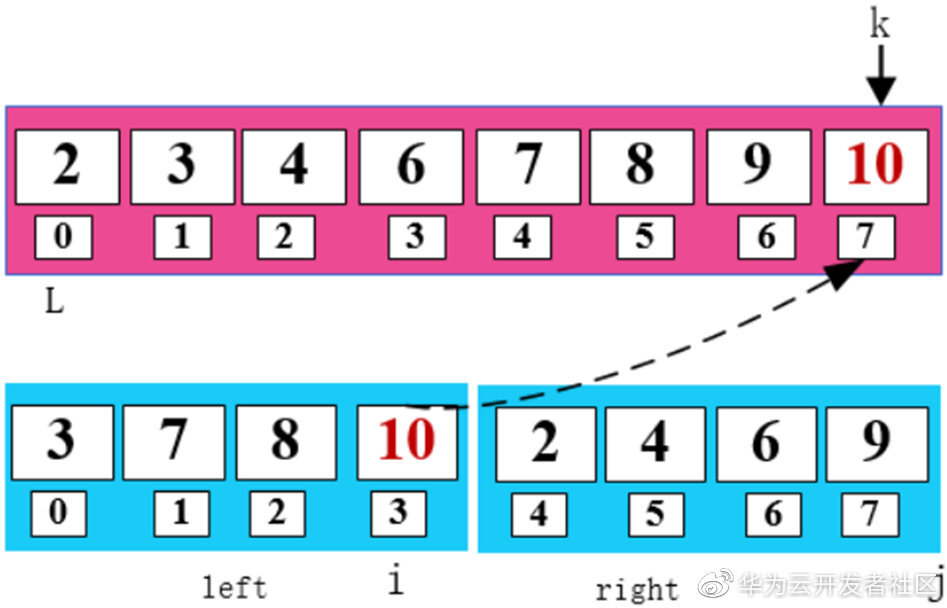​一顿操作猛如虎，这样就把两个有序的数组通过归并的方式排好顺序啦，是不是很赞。

## 四、问题解决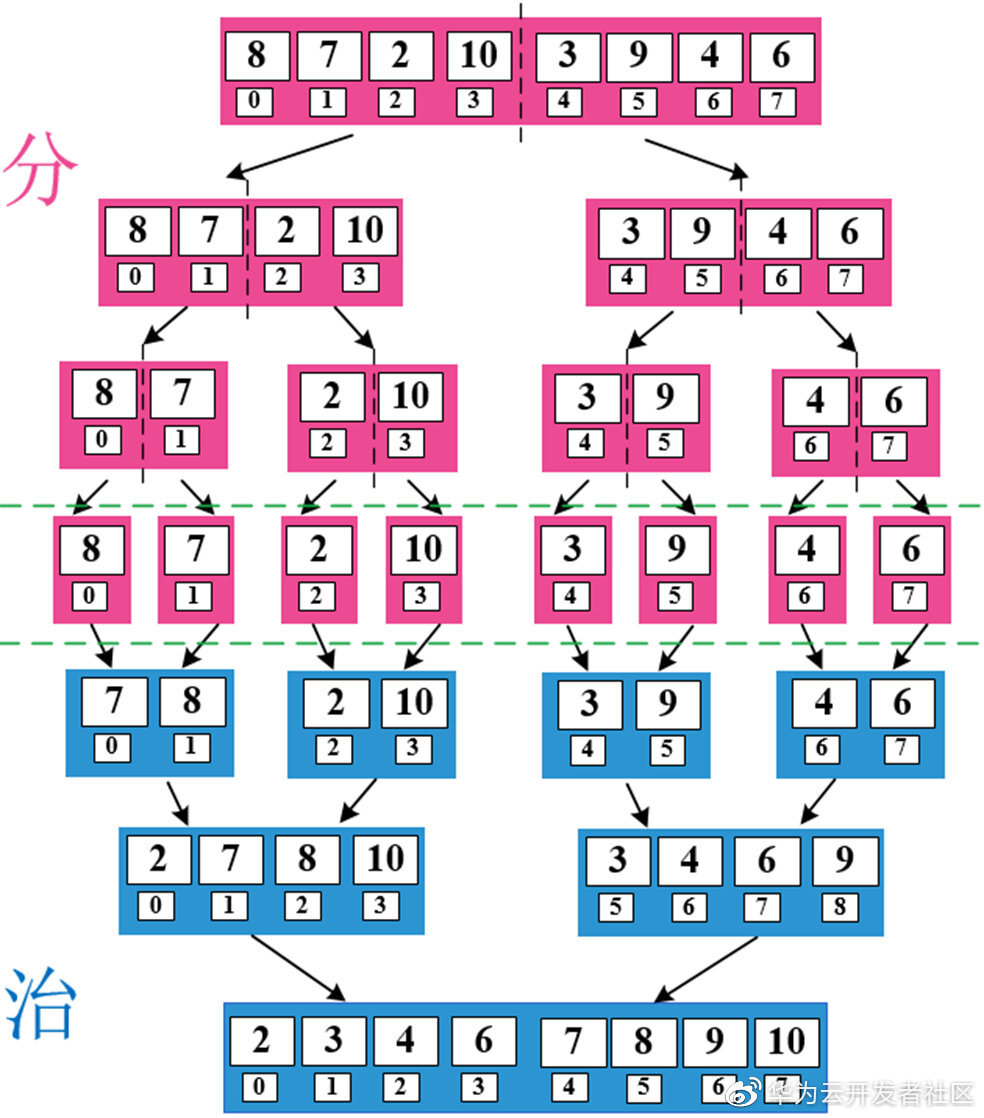## 五、算法实现

#include <stdio.h>       void merge(int arr[], int L, int M, int R) {         int LEFT_SIZE = M - L;         int RIGHT_SIZE = R - M + 1;         int left[LEFT_SIZE];         int right[RIGHT_SIZE];         int i, j, k;         // 填充左边的数组          for (i=L; i<M; i++){            left[i-L] = arr[i];          }         // 填充右边的数组          for (i=M; i<=R; i++){            right[i-M] = arr[i];          }       // for (int i=0; i<LEFT_SIZE; i++){       // printf("%d\n",left[i]);       // }       //        // for (int i=0; i<RIGHT_SIZE; i++){       // printf("%d\n",right[i]);       // }         // 合并数组          i = 0; j = 0; k = L;          while (i < LEFT_SIZE && j < RIGHT_SIZE){            if (left[i] < right[j]){              arr[k] = left[i];              i++;              k++;            }else{              arr[k] = right[j];              j++;              k++;            }          }          while(i < LEFT_SIZE){            arr[k] = left[i];            i++;            k++;          }          while(j < RIGHT_SIZE){            arr[k] = right[j];            j++;            k++;          }       }       void mergeSort(int arr[], int L, int R){         if (L == R){           return;         }else{           int M = (L + R) / 2;           mergeSort(arr,L,M);           mergeSort(arr, M+1,R);           merge(arr, L, M+1,R);         }       }       int main(){       // int arr[] = {3,7,8,10,2,4,6,9};         int arr[] = {8,7,2,10,3,9,4,6};         int L = 0;         int M = 4;         int R = 7;         mergeSort(arr,L,R);         for (int i=0; i<=R; i++){           printf("%d\n",arr[i]);         }       }

​输出：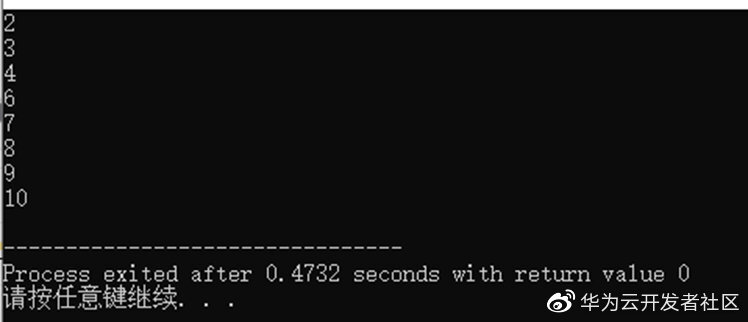## 七、适用场景## 评论